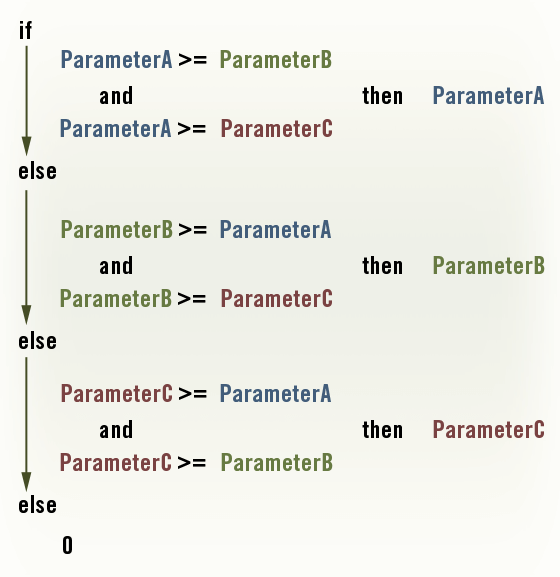# Revit Families 402 – Greater Than or Equal To

• Posted on April 13, 2011

As discussed in “Revit Families 103 – Formula Basics” there is no native function in Revit for Greater Than or Equal to (>=) and it’s brother Less Than or Equal to (<=). That’s no problem. With the basic conditional statements we can recreate them.

## Greater Than or Equal to (>=)

What we want to do:

If (ParameterA >= ParamaterB, <true>, <false>)

How we do it:

If(not(ParameterA < ParamaterB), <true>, <false>)

## Less Than or Equal to (<=)

What we want to do:

If (ParameterA <= ParamaterB, <true>, <false>)

How we do it:

If(not(ParameterA > ParamaterB), <true>, <false>)

## Find the Greatest of Three Values

Now that we know how to do greater than or equal to, what if you have three parameters and you want to know the largest of them?

ParamaterA
ParameterB
ParameterC

What we want to do:

Return the largest number, ParameterA, ParamaterB, or ParamaterC

How we do it:

if( and( not( ParameterA < ParameterB), not(ParameterA < ParameterC)), ParameterA, if( and( not( ParameterB < ParameterA), not( ParameterB < ParameterC)), ParameterB, if( and( not( ParameterC < ParameterA), not( ParameterC < ParameterB)), ParameterC, 0)))

The “0” at the end should be replaced with a value that has the same units as your other parameters. ie. feet, mm, etc.

I would just copy and paste that into my family but let me make that easier to understand:As you can see the logic is pretty straight forward, the >= are taken from what we learned at the top of this post (we do the logical inverse: if it’s not less than). Now that you understand it you can easily find the largest value of two, three, or one hundred different values! Hopefully, someday, Revit will just include a nice easy way to do this.